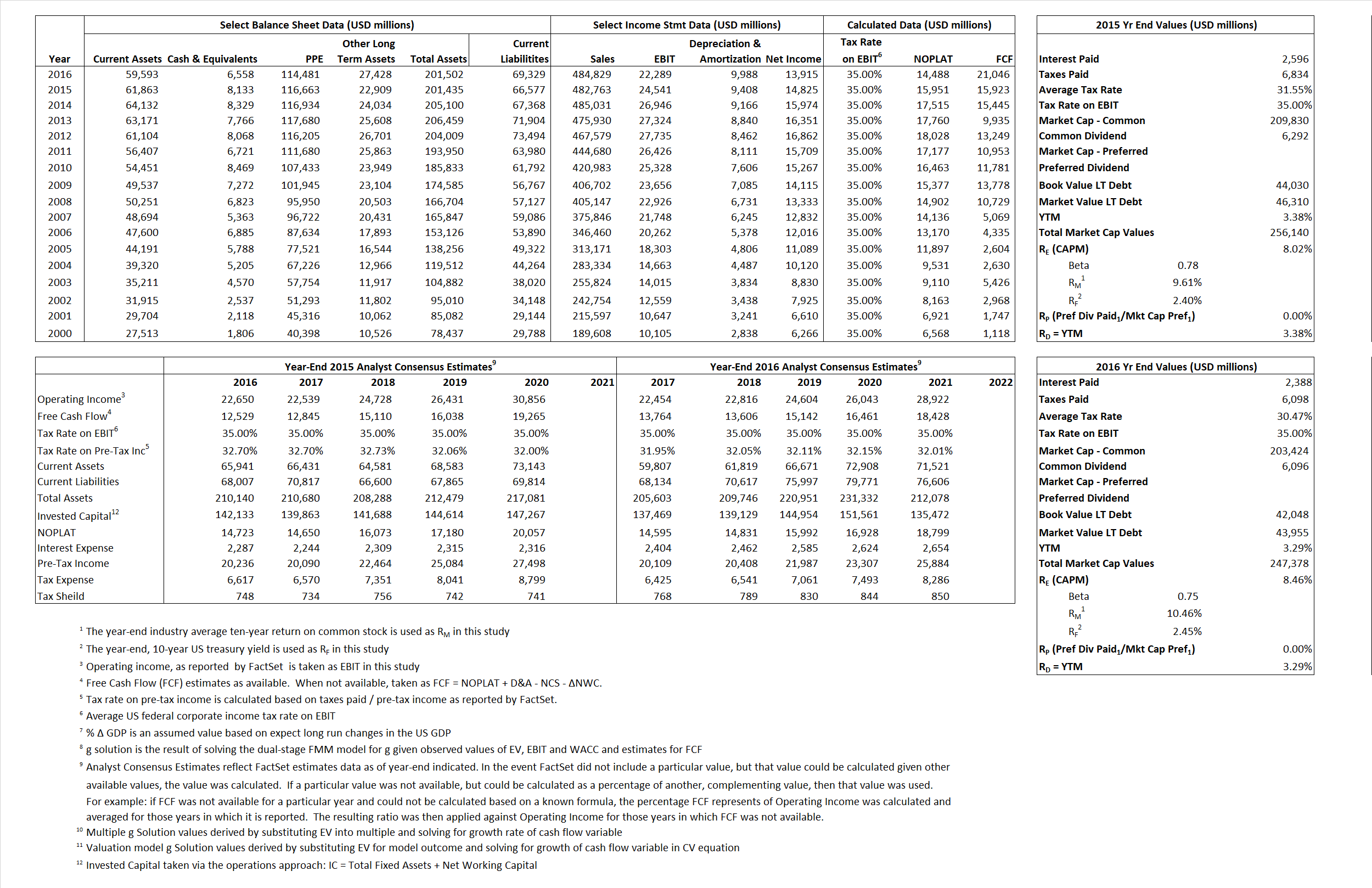# Wal-Mart

## Analyst Listing

The following analysts provide coverage for the subject firm as of May 2016:

 Broker Analyst Analyst Email Stephens Inc Ben Bienvenu ben.bienvenu@stephens.com Raymond James Budd Bugatch budd.bugatch@raymondjames.com Northcoast Research Chuck Cerankosky chuck.cerankosky@northcoastresearch.com Jefferies Daniel Binder dbinder@jefferies.com Consumer Edge Research David A. Schick dschick@consumeredgeresearch.com Evercore ISI Greg Melich greg.melich@evercoreisi.com Buckingham Research John Zolidis jzolidis@buckresearch.com Telsey Advisory Group Joseph Feldman jfeldman@telseygroup.com Daiwa Securities Co. Ltd. Kahori Tamada kahori.tamada@us.daiwacm.com Credit Suisse Michael Exstein michael.exstein@credit-suisse.com Cowen & Company Oliver Chen oliver.chen@cowen.com Deutsche Bank Research Paul Trussell paul.trussell@db.com CRT Capital Group Renato Basanta rbasanta@sterneageecrt.com Nomura Research Robert S. Drbul robert.drbul@nomura.com RBC Capital Markets Scot Ciccarelli scot.ciccarelli@rbccm.com Wolfe Research Scott Mushkin smushkin@wolferesearch.com BMO Capital Markets Wayne Hood wayne.hood@bmo.com

## Primary Input Data## Derived Input Data

### Equational Form

Net Operating Profit Less Adjusted Taxes NOPLAT  15,951 14,488$NOPLAT\, =\, EBIT\, x\, (1 \,-\, Avg \,\,Tax\,\, Rate\,\, on\,\, EBIT)$
Free Cash Flow FCF 15,923 21,046$FCF\,=NOPLAT\,+\,Non-Cash\,Expenses-\Delta NWC\,-\,NCS$
Tax Shield TS 819 728$TS\,=\,Interest\,\,Paid\,\,x\,\, Avg \,\,Tax\,\,Rate\,\, on\,\, Pre-Tax\,\, Income$
Invested Capital IC 134,859 132,174$IC\,=\,Fixed\,\,Operating\,\,Assets\,\,+\,\,Net\,\, Working\,\, Capital$
Return on Invested Capital ROIC 11.83% 10.96%$ROIC\,=\,\frac { NOPLAT }{ IC }$
Net Investment NetInv 6,534 7,303$NetInv\,=\,{ {IC}_{1}}-{{IC}_{0}}+Depreciation$
Investment Rate IR 40.96% 50.41%$IR\,=\,\frac {NetInv}{NOPLAT}$
Weighted Average Cost of Capital
WACCMarket 6.99% 7.36%$WACC\,=\,\frac { E }{ V } { R }_{ E }\,+\,\frac { P }{ V } { R }_{ P }\,+\,\frac { D }{ V } { R }_{ D }\left( 1- Avg\,\, Tax\,\,Rate\,\,on\,\,Pre-Tax\,\,Income \right)$
WACCBook  6.39%  6.14%
Enterprise value
EVMarket 248,007 240,821$EV\,=\,Market\,\,Cap\,\,Equity\,+\,\,Long\,\,Term\,\,Debt\,-\,Cash$
EVBook  247,302  238,914
Long-Run Growth
g = IR x ROIC
4.85% 5.53% Long-run growth rates of the income variable are used in the Continuing Value portion of the valuation models.
g = %$\Delta$ GDP  2.50%  2.50%
Margin from Operations M 5.08% 4.60%$M\,\,=\,\,\frac{EBIT}{SALES}$
Depreciation/Amortization Rate D 27.71% 30.95%$D\,\,=\,\,\frac{D+A}{EBITDA}$

## Valuation Multiple Outcomes

The outcomes presented in this study are the result of original input data, derived data, and synthesized inputs.

### model g solution

12/31/2015 12/31/2016 12/31/2015 12/31/2016 12/31/2015 12/31/2016

EV/SALES$\frac {EV}{Sales} \,= \,\frac{ROIC\, -\, g}{ROIC\,(WACC\,-\,g)}\,(1\,-\,T)\,(M)$

0.51  0.50  1.23%  2.98%  1.85%  5.11%

EV/EBITDA$\frac {EV}{EBITDA} \,= \,\frac{ROIC\, -\, g}{ROIC\,(WACC\,-\,g)}\,(1\,-\,T)\,(1\,-\,D)$

7.31 7.46 1.23% 2.98% 1.85% 5.11%

EV/NOPLAT$\frac {EV}{NOPLAT} \,= \,\frac{ROIC\, -\, g}{ROIC\,(WACC\,-\,g)}$

15.55 16.62 1.23% 2.98% 1.85% 5.11%

EV/FCFOPS$\frac {EV}{FCF_{OPS}} \,= \,\frac{ROIC\, -\, g}{ROIC\,(WACC\,-\,g)}\,(1\,-\,T)$

15.58 11.44 1.23% 2.98% 1.85% 5.11%

EV/EBIT$\frac {EV}{EBIT} \,= \,\frac{ROIC\, -\, g}{ROIC\,(WACC\,-\,g)}\,(1\,-\,T)$

10.11 10.80 1.23% 2.98% 1.85% 5.11%

EV/IC$\frac {EV}{IC} \,= \,\frac{ROIC\, -\, g}{WACC\,-\,g}$

1.84 1.82 1.23% 2.98% 1.85% 5.11%